Question

# A certain state has the highest high school graduation rate of all states at 85%. A....

A certain state has the highest high school graduation rate of all states at 85%.

A. In a random sample of 10 high school students from the state, what is the probability that 8 will graduate?

B. In a random sample of 10 high school students from the state, what is the probability than 7 or fewer will graduate?

C. What is the probability that at least 8 high school students in our sample of 10 will graduate?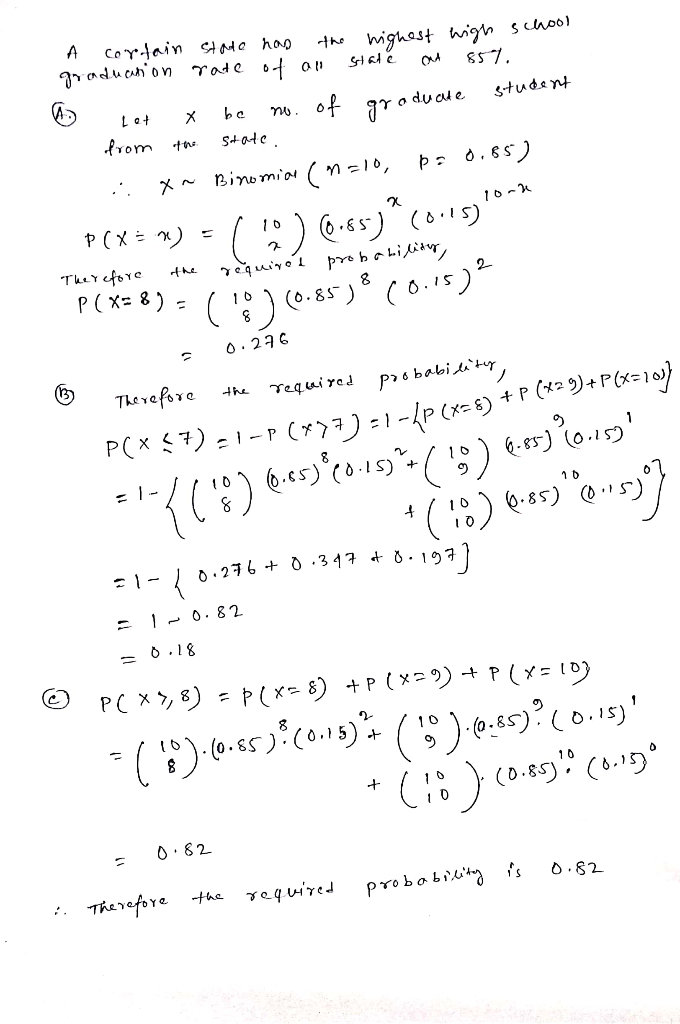#### Earn Coins

Coins can be redeemed for fabulous gifts.

Similar Homework Help Questions
• ### Approximately 10.3% Of American High School Students Drop Out Before Graduation

Approximately 10.3% Of American High School Students Drop Out Before Graduation. Choose 10 Students Entering High School At Random. Find the probability that at least 6 will graduate

• ### Approximately 10.3% of American high school students drop out of school before graduation. Assume the variable...

Approximately 10.3% of American high school students drop out of school before graduation. Assume the variable is binomial. Choose 10 students entering high school at random. Find the probabilities. Round the answers to at least four decimal places. (a) No more than 2 drop out = (b) At least 6 = graduate = (c) All 10 stay in school and graduate =

• ### Approximately 10% of high school students drop out of school before graduation. Choose 10 students entering...

Approximately 10% of high school students drop out of school before graduation. Choose 10 students entering high school at random. Find(a) the probability that all 10 drop out (b) the probability that no more than 1 drop out (c) the mean and standard deviation of the number of high school students drop out of school before graduation

• ### The data shows a sample of states' percentage of public high school graduation rates for a...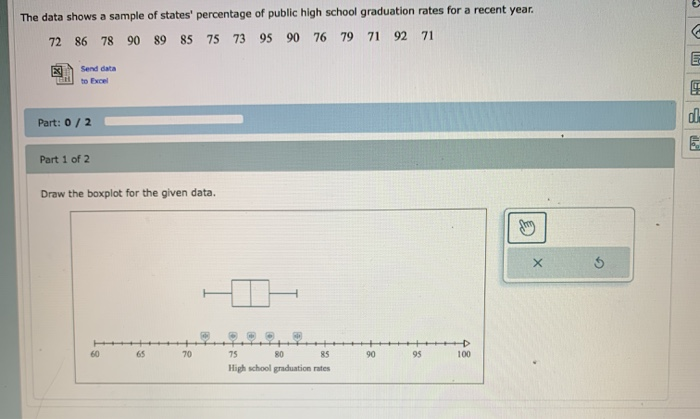The data shows a sample of states' percentage of public high school graduation rates for a recent year. 72 86 78 90 89 85 75 73 95 90 76 79 71 92 71 3. Send Part: 0/2 Part 1 of 2 Draw the boxplot for the given data. High school graduation rates

• ### Question 28 3 pts Suppose that the graduation rate in a school district is 85 percent....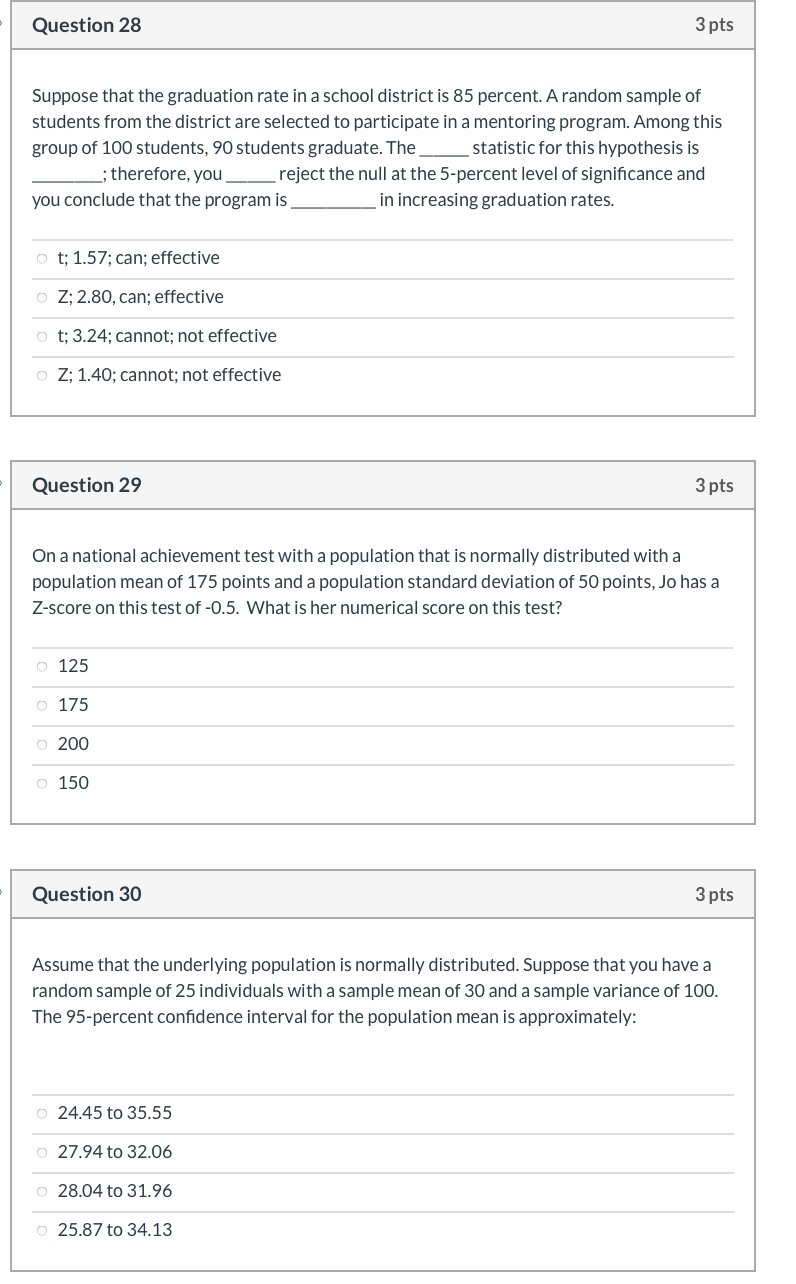Question 28 3 pts Suppose that the graduation rate in a school district is 85 percent. A random sample of students from the district are selected to participate in a mentoring program. Among this group of 100 students, 90 students graduate. The statistic for this hypothesis is ; therefore, you reject the null at the 5-percent level of significance and you conclude that the program is in increasing graduation rates. ot; 1.57; can; effective o Z; 2.80, can; effective t;...

• ### Assume all variables are binomial. (Note: If values are not found in Table B of Appendix...

Assume all variables are binomial. (Note: If values are not found in Table B of Appendix C, use the binomial formula.)High School Dropouts Approximately 10.3% of American high school students drop out of school before graduation. Choose 10 students entering high school at random. Find the probability thata. No more than two drop outb. At least 6 graduatec. All 10 stay in school and graduateSource: www.infoplease.com

• ### U.S. schools face an ongoing challenge: as students get closer to high school graduation, their enthusiasm...

U.S. schools face an ongoing challenge: as students get closer to high school graduation, their enthusiasm for school falls. A Gallup poll in June 2017 has discovered that fifth graders are most engaged with school, while 11th graders are least. The latest Gallup poll showed that only 32% of 11th graders are engaged in school. Assume this value represents the population proportion. Suppose we randomly sample 150 11th graders in the U.S. and ask them their engagement in school. ?...

• ### Tulane reports an overall 4 year graduation rate of 75%. From a random sample of 8...

Tulane reports an overall 4 year graduation rate of 75%. From a random sample of 8 students, use the Binomial Distribution to answer the following: a. What is the probability that 4 or more will graduate in 4 years?

• ### 3. A study of community college enrollments in a certain state finds that 60% of all...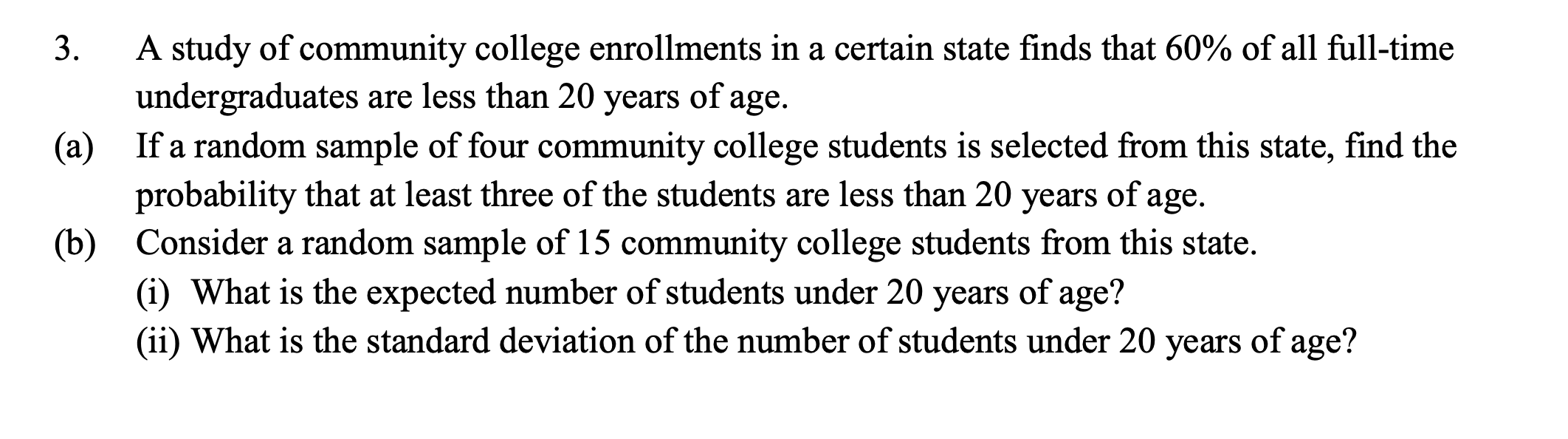3. A study of community college enrollments in a certain state finds that 60% of all full-time undergraduates are less than 20 years of age. If a random sample of four community college students is selected from this state, find the probability that at least three of the students are less than 20 years of age. Consider a random sample of 15 community college students from this state. (i) What is the expected number of students under 20 years of...

• ### The Statistical Abstract of the United States reported that 66% of students who graduated from high...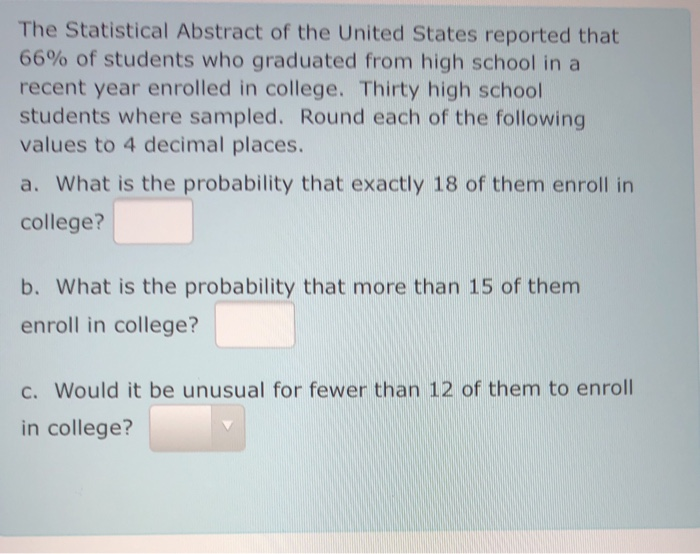The Statistical Abstract of the United States reported that 66% of students who graduated from high school in a recent year enrolled in college. Thirty high school students where sampled. Round each of the following values to 4 decimal places. a. What is the probability that exactly 18 of them enroll in college? b. What is the probability that more than 15 of them enroll in college? c. Would it be unusual for fewer than 12 of them to enroll...